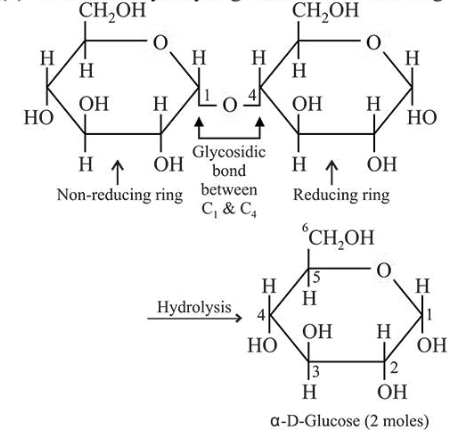# Two monomers in maltose are:Question:

Two monomers in maltose are:

1. $\alpha-D-g l u c o s e$ and $\beta-D-g l u c o s e$

2. $\alpha-\mathrm{D}-\mathrm{glucose}$ and $\alpha-\mathrm{D}$-galactose

3. $\alpha-\mathrm{D}-\mathrm{glucose}$ and $\alpha-\mathrm{D}$-fructose

4. $\alpha-\mathrm{D}-\mathrm{glucose}$ and $\alpha-\mathrm{D}-\mathrm{glucose}$

Correct Option: , 4

Solution:

Maltose on hydrolysis gives two moles of $\alpha$-D-glucose.